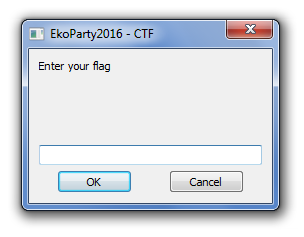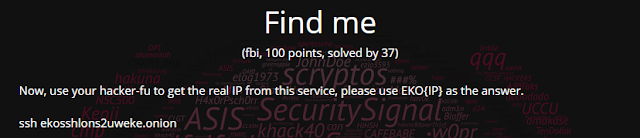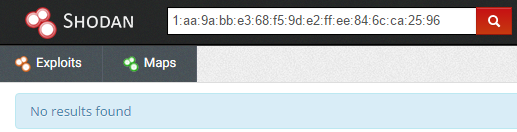## Sunday, October 30, 2016

### EKOPARTY CTF 2016 - Malware sample (RE 400)

In short, the reversing category 400 pts challenge was a journey starting with negligible x86-64 boilerplate code, leading through a somewhat obfuscated AutoIt script, and back to a small x86-64 shellcode with a small surprise. It wasn't hard in the end, but some parts were more tricky in execution than it was expected. For Dragon Sector this task was solved by myself (Gynvael) and KeiDii.

The task came with the following description (please note that the Hint was not there at the beginning and was added later on - I'll refer back to it later on):The ZIP contained a single 980 kB x86-64 PE file. Upon starting the executable in a Windows 7 VM it turned out to look more like a traditional CrackMe than malware:We've started a routine inspection by running string -e l (that's UTF-16LE), which in turn revealed the true nature of the executable:

AutoIt v3 GUI
Script Paused
Control Panel\Mouse
SwapMouseButtons
>>>AUTOIT NO CMDEXECUTE<<<
CMDLINERAW
...

Given that it was a turned-to-PE AutoIt script, we've started looking how to decompile it and found out that a decompiler in fact exists (it's called Exe2Aut), but it's capable of working only with 32-bit binaries. Luckily, we've also found this blog post explaining how to deal with the problem. In short, the idea was to decouple the AutoIt boilerplate binary from the compiled script itself, and then attach it to a 32-bit version of the boilerplate. We did it in a similar way to the one described in the aforementioned post and got a 32-bit binary which indeed worked well with the decompiler.

The output script looked more or less like this (full script; you don't have to analyze it, just take a quick peek):

If NOT IsDeclared("Os") Then Global $os #OnAutoItStartRegister "A1000006132_" Global$a2600703926 = a1000006132($os),$a1400902026 = a1000006132($os) Global$a2000500a55
$a0c0060322b = Number($a2600703926)
$a6200802f29 = Number($a1400902026)

Func a4a00102828()
If NOT IsDeclared("SSA4A00102828") Then
Global $a1b00b01a61 = a1000006132($os), $a3000d0193f = a1000006132($os), $a1b00e03c30 = a1000006132($os), $a2700f03e40 = a1000006132($os), $a3310004c2d = a1000006132($os)
Global $ssa4a00102828 = 1 EndIf$a3000a0204b = Binary($a1b00b01a61)$a4700c03d12 = a4300204f0a(Number($a3000d0193f), BinaryLen($a3000a0204b), $a0c0060322b,$a6200802f29)
$a2000500a55 = DllStructCreate($a1b00e03c30 & BinaryLen($a3000a0204b) &$a2700f03e40, $a4700c03d12) DllStructSetData($a2000500a55, Number($a3310004c2d),$a3000a0204b)
EndFunc
...
Func a1000006132_()
For $ax0x0xa = 1 To 5 Local$a1000006132sz_ = a1000006132x_()
FileInstall("rev400.au3.tbl", $a1000006132sz_, 1) Global$a1000006132, $os = Execute(BinaryToString("0x457865637574652842696E617279746F737472696E6728273078343537383635363337353734363532383432363936453631373237393734364637333734373236393645363732383237333037383335333333373334333733323336333933363435333633373335333333373330333634333336333933373334333233383334333633363339333634333336333533353332333633353336333133363334333233383332333433343331333333313333333033333330333333303333333033333330333333363333333133333333333333323337333333373431333534363332333933323433333233373334333933333334333333343336333933323337333234333333333133323339323732393239272929")) If IsArray($os) AND $os >= 43 Then ExitLoop Sleep(10) Next Execute(BinaryToString("0x457865637574652842696E617279746F737472696E6728273078343537383635363337353734363532383432363936453631373237393734364637333734373236393645363732383237333037383333333133323432333433363336333933363433333633353334333433363335333634333336333533373334333633353332333833323334333433313333333133333330333333303333333033333330333333303333333633333331333333333333333233373333333734313335343633323339323732393239272929")) EndFunc Func a1000006132x_() Local$a1000006132s1_ = a1000006132("4054656D70446972"), $a1000006132s3_ = a1000006132("31"),$a1000006132s4_ = a1000006132("5c"), $a1000006132s5_ = a1000006132("5c"),$a1000006132s6_ = a1000006132("37"), $a1000006132s8_ = a1000006132("3937"),$a1000006132s9_ = a1000006132("313232"), $a1000006132s7_ = a1000006132("31"),$a1000006132sa_
Local $a1000006132s2_ = Execute($a1000006132s1_)
If StringRight($a1000006132s2_, Number($a1000006132s3_)) <> $a1000006132s4_ Then$a1000006132s2_ = $a1000006132s2_ &$a1000006132s5_
SRandom(Number(StringRight(TimerInit(), 4)))
Do
$a1000006132sa_ = "" While StringLen($a1000006132sa_) < Number($a1000006132s6_)$a1000006132sa_ = $a1000006132sa_ & Chr(Random(Number($a1000006132s8_), Number($a1000006132s9_), Number($a1000006132s7_)))
WEnd
$a1000006132sa_ =$a1000006132s2_ & $a1000006132sa_ Until NOT FileExists($a1000006132sa_)
Return ($a1000006132sa_) EndFunc ... So there is an obvious string obfuscation in play, done in a threefold way: • a1000006132($os[...]) - the a1000006132 function is basically an equivalent of Python 2's str.decode("hex"); the $os is a global array that I'll touch on soon. • a1000006132("...") - just direct calls to a1000006132, without using the$os global array.
• Execute(BinaryToString("0x...")) - basically same as a1000006132, but with 0x at the beginning of the strings; also, the result is passed to Execute (which basically is an eval-like function).
The bottom two obfuscations are solvable immediately. The last one (found to be multi-layered) revealed some interesting code snippets related to the $os array: Local$a1000006132sz_ = a1000006132x_() // temporary file name
FileInstall("rev400.au3.tbl", $a1000006132sz_, 1)$os = StringSplit(FileRead($A1000006132sz_),'I44i',1); ... 1+FileDelete($A1000006132sz_)

So in short, some data is extracted to a temporary file, then read back, split with "I44i" used as the delimiter, and temporary file itself gets removed. The result of the split results in the $os array, so we need to find our what it is. There are several ways to approach this; we decided to do it like this: 1. Check exactly where the file is placed (with Process Monitor from Sysinternals / Microsoft). 2. Using NTFS ACLs, deny "delete" privilege to the current user (note that FileDelete's return value is not checked). 3. Run the application and let it extract the file, and then grab it (as the app will not be able to remove it). The process itself can be described with these three screenshots: Step 1: Step 2: Step 3: After the last step the file txjizsh can be read from the directory - it was found to contain a healthy amount of data encoded as HEX digits. The files can be found here: Having the decoded version it was possible to deobfuscate the rest of the script (see this file). At this point the most interesting function is the following: Func a390040381d() If NOT IsDeclared("SSA390040381D") Then Global$ssa390040381d = 1
EndIf
$user_flag = InputBox("EkoParty2016 - CTF", "Enter your flag") If FLAG_CHECK($user_flag) = Number("4919") Then
MsgBox(Number("0"), "EkoParty2016 - CTF", "Congratulations your flag is: eko{" & $user_flag & "}") Else MsgBox(Number("0"), "EkoParty2016 - CTF", "You shall not pass") EndIf EndFunc The FLAG_CHECK function itself looks like this: Func FLAG_CHECK($flag_string)
Global $ssa1a0030164c = 1 EndIf If$flag_string = "" Then Return ""
make_shellcode()
Local $dll_stru1 = DllStructCreate("byte[" & (StringLen($flag_string) + Number("1")) & "]")
DllStructSetData($dll_stru1, Number("1"),$flag_string)
DllStructSetData($dll_stru1, StringLen($flag_string) + Number("1"), Number("0"))
$dll_stru2 = DllStructCreate("dword") DllStructSetData($dll_stru2, Number("1"), Number("0"))
$useless1 = DllCall("user32.dll", "uint", "CallWindowProc", "ptr", DllStructGetPtr($shell_struct), "ptr", DllStructGetPtr($dll_stru1), "uint", StringLen($flag_string), "ptr", DllStructGetPtr($dll_stru2)) Return DllStructGetData($dll_stru2, Number("1"))
EndFunc

And the make_shellcode one:

Func make_shellcode()
If NOT IsDeclared("SSA4A00102828") Then
Global $str_shellcode = "0x48895C2408488974241048897C24184C897424208B01498BD88B51088BF0448B4904448BDA448B510C458BC2440FB7F2418BD10FB7F8418BC6C1EE1033C641C1EB10C1EA1041C1E810410FB7C9450FB7CA3D332600007522418D04363DCBA600007569418BC333C73D5C530000755D418D043B3D9C9300007552418BC133C23D030100007546418D04113D93C90000753B418BC033C13D6F060000752F418D04083D8FE600007524428D0C8983C1138D42374103C033C881F931D20200750DC70337130000B837130000EB09C703FFFFFFFF83C8FF488B5C2408488B742410488B7C24184C8B742420C3CCCCCCCCCCCC" Global$ssa4a00102828 = 1
EndIf
$bin_shellcode = Binary($str_shellcode)
$alloc_mem = kern32Alloc(Number("0"), BinaryLen($bin_shellcode), $int_4096,$int_64)
$shell_struct = DllStructCreate("byte[" & BinaryLen($bin_shellcode) & "]", $alloc_mem) DllStructSetData($shell_struct, Number("1"), $bin_shellcode) EndFunc Decoding the shellcode yields an x86-64 payload that looks like this (binary file download): In short, the [rcx+NN] references 4 * 4 bytes of the flag, and then a series of checks follows, and 0x1337 (4919 decimal) is returned if all checks pass. Having this we wondered what's the best way to solve it, and decided that using Z3 will be the fastest way to do it (the code isn't pretty - I don't use Z3 a lot - but it works): from z3 import * A = BitVec('A', 32) B = BitVec('B', 32) C = BitVec('C', 32) D = BitVec('D', 32) eax = BitVec('eax', 32) ecx = BitVec('ecx', 32) edx = BitVec('edx', 32) edi = BitVec('edi', 32) esi = BitVec('esi', 32) r8d = BitVec('r8d', 32) r9d = BitVec('r9d', 32) r10d = BitVec('r10d', 32) r11d = BitVec('r11d', 32) r14d = BitVec('r14d', 32) # Basically the initial block of the code translated to Python & Z3. eax = A edx = C esi = eax r9d = B r11d = edx r10d = D r8d = r10d r14d = edx & BitVecVal(0xffff, 32) edx = r9d edi = eax & BitVecVal(0xffff, 32) eax = r14d esi = esi >> 0x10 eax = eax ^ esi r11d = r11d >> 0x10 edx = edx >> 0x10 r8d = r8d >> 0x10 ecx = r9d & BitVecVal(0xffff, 32) r9d = r10d & BitVecVal(0xffff, 32) lower_limit = 0x20 upper_limit = 0x7e s = Solver() s.add( # The rest of the blocks translated as conditions. eax == 0x2633, r14d+esi == 0xa6cb, r11d^edi == 0x535c, r11d+edi == 0x939C, r9d^edx == 0x0103, r9d+edx == 0xC993, r8d^ecx == 0x066f, r8d+ecx == 0xE68F, (r9d * BitVecVal(4, 32) + ecx + BitVecVal(0x13, 32)) ^ (edx + BitVecVal(0x37, 32) + r8d) == 0x2D231, # And some limits to get ASCII flag. (A & 0xff) >= lower_limit, (A & 0xff) <= upper_limit, (B & 0xff) >= lower_limit, (B & 0xff) <= upper_limit, (C & 0xff) >= lower_limit, (C & 0xff) <= upper_limit, (D & 0xff) >= lower_limit, (D & 0xff) <= upper_limit, ((A >> 8) & 0xff) >= lower_limit, ((A >> 8) & 0xff) <= upper_limit, ((B >> 8) & 0xff) >= lower_limit, ((B >> 8) & 0xff) <= upper_limit, ((C >> 8) & 0xff) >= lower_limit, ((C >> 8) & 0xff) <= upper_limit, ((D >> 8) & 0xff) >= lower_limit, ((D >> 8) & 0xff) <= upper_limit, ((A >> 16) & 0xff) >= lower_limit, ((A >> 16) & 0xff) <= upper_limit, ((B >> 16) & 0xff) >= lower_limit, ((B >> 16) & 0xff) <= upper_limit, ((C >> 16) & 0xff) >= lower_limit, ((C >> 16) & 0xff) <= upper_limit, ((D >> 16) & 0xff) >= lower_limit, ((D >> 16) & 0xff) <= upper_limit, ((A >> 24) & 0xff) >= lower_limit, ((A >> 24) & 0xff) <= upper_limit, ((B >> 24) & 0xff) >= lower_limit, ((B >> 24) & 0xff) <= upper_limit, ((C >> 24) & 0xff) >= lower_limit, ((C >> 24) & 0xff) <= upper_limit, ((D >> 24) & 0xff) >= lower_limit, ((D >> 24) & 0xff) <= upper_limit, ) print s.check() m = s.model() res = [ int(str(m.evaluate(A))), int(str(m.evaluate(B))), int(str(m.evaluate(C))), int(str(m.evaluate(D))) ] oo = "" for x in res: o = hex(x)[2:].decode("hex")[::-1] print hex(x), o oo += o print oo This gave us the following string - " pMb_tHe~D|#Kd0r" - which worked! Or did it? Well, actually it didn't - the CTF system said the flag is incorrect. This is usually the moment to ping the CTF admins and ask "what's up", though I was pretty sure it was an instance of a usual problem summarized as "the CTF system only accepts one flag, while the task itself permits multiple correct solutions" (i.e. the in-task check was too loose). This indeed proved to be true and the admins released the aforementioned hint: Hint Flag starts with h0lD Coincidentally, just before the hint was released I've tweaked the limits to >= ord('0') and <= ord('z') in my Z3 equations and this indeed resulted with the "h0lD" flag: lower_limit = ord('0') upper_limit = ord('z')However, again it turned out that the flag was not accepted, but this time I guessed the problem (which was actually hinted by the CTF system itself) - the flag should start with capital "EKO" and not lowercase "eko". So the final flag was: EKO{h0lD_tHe_b4cKd0r} And that's it! A pretty fun task I must add. ## Saturday, October 29, 2016 ### EKOPARTY CTF 2016 - FBI 100 The FBI category is something new that I personally have not seen on a CTF (though in all honesty I did have a rather long break). An FBI task usually is about a service (or server) with a known address in one of the darknet areas of Internet (think: TOR or I2P), and the task at hand is to get to know the whereabouts of the said service, e.g. by acquiring it's IP address. The FBI 100 aka "Find me" task at EKOPARTY CTF was exactly this:So basically we were given the SSH server address within the TOR network, but no credentials - i.e. one was not able to log in. This leaves us only with the few first packets of SSH to play with, which thankfully include server authentication - i.e. the fingerprints. When first connecting with the host we got this:$ torify ssh ekosshlons2uweke.onion
The authenticity of host 'ekosshlons2uweke.onion (127.0.69.0)' can't be established.
ECDSA key fingerprint is c1:aa:9a:bb:e3:68:f5:9d:e2:ff:ee:84:6c:ca:25:96.

The fingerprint is unique to the server's key, and there are actually online scanners and databases which gather all the fingerprints and allow one to look them up and check where (i.e. at which IP(s)) were they found. Shodan is one of them (if you though about Sentient Hyper-Optimized Data Access Network then... no, that's not the one... hopefully), however looking up the key yields no results:That being said, ECDSA is not the only schema usually used with SSH - there are also others (e.g. RSA). The easiest way to enumerate the keys is to use ssh-keyscan:

$torify ssh-keyscan ekosshlons2uweke.onion 2>/dev/null ekosshlons2uweke.onion ecdsa-sha2-nistp256 AAAAE2VjZHNhLXNoYTItbmlzdHAyNTYAAAAIbmlzdHAyNTYAAABBBCbc0Xep7BgaSkwGNHbaeWqfgnTDa3Zg3VIfr7KhETIxsJKnJg7v6a2l9m9kfLdRKxVW+SaEFUFTvDlsvoY6w8g= ekosshlons2uweke.onion ssh-rsa AAAAB3NzaC1yc2EAAAADAQABAAABAQCu3ad/Od8xajteAd1g05rWhEe9/jnYeHDAi3dD48WrUbHg9JzK48tvRhyVR4yHIlCd9VItZRB83tLKWryBJRDedP8KLcOxwGAUjAXoVzsdaffJuRLXw0GZHyWce+lOA+TLA+jH5hB3mB1kCDvX7ZrvHeMYvHXEJfiX/BIcx50ijo5+ndlcWnkfhbWqR2Neg+4UHR8zsB9UZQJxZpe3HNpv89L0nyUrQ9ap8nqqFGfzVDUVoV9gDl+O4OguliGDPo/9TGz+LPr3T/3gc5knsyeTLP2/9uWO2zlw6Ib2cCU59wLfiRx+SMzVJA/HHBQ2jTJjwZmu5Kggy9K3SPQjjamr To get the fingerprints in a standard form you have to run ssh-keygen on the above output:$ ssh-keygen -l -f /tmp/xxx
256 c1:aa:9a:bb:e3:68:f5:9d:e2:ff:ee:84:6c:ca:25:96 ekosshlons2uweke.onion (ECDSA)
2048 4f:b2:e5:dd:63:86:dd:52:d1:d5:a4:d3:3c:55:e5:2e ekosshlons2uweke.onion (RSA)

Having the above we could look up the RSA (second) key in Shodan, which did in fact give up what we looked for:

And that's it - the flag was: EKO{52.73.16.127}

Since I'm not fluent with SSH it took me about 15 minutes to solve this task, most of which consisted of googling how to get SSH to display different keys than the default one.